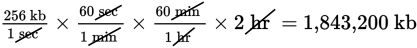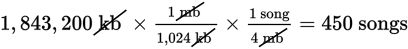# SAT Math Multiple Choice Question 830: Answer and Explanation

### Test Information

Question: 830

5. A typical song downloaded from the Internet is 4 megabytes in size. Lindy has satelliteInternet, and her computer downloads music at a rate of 256 kilobytes per second.If 1 megabyte equals 1,024 kilobytes, about how many songs can Lindy download in 2hours?

• A. 128
• B. 450
• C. 1,800
• D. 1,920

Explanation:

B

Difficulty: Easy

Category: Problem Solving and Data Analysis / Rates, Ratios, Proportions, and Percentages

Strategic Advice: When converting lots of units, use the factor-label method and let the unit conversions guide you to the correct answer.

Getting to the Answer: The initial rate is given in kilobytes per second, the size per song is given in megabytes, and the question asks for the number of songs that can be downloaded in 2 hours. Arrange the given rates so that the units in the end result are what you're looking for: